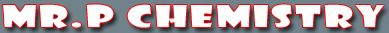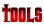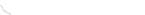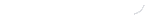activity seriesbond energybond lengthscalculatorcommon namesconversion factors: energyconversion factors: lengthconversion factors: massconversion factors: pressureconversion factors: temp.conversion factors: volumecovalent prefixesdensity solverdiatomic elementse-config. chartelement listgas law formulasKa's of polyprotic acidsKa's of weak acidsKb's of weak basesmetric conversion chartmole conversion chartmolecular geometriesorganic prefixesperiodic tableperiodic table (flash)pH/pOH converterpolyatomic ionspressure converterSI unitssolubility chartsolubility of salts rulessolubility product constantsstoichiometry charttemp. conversiontemp. formulasthermodynamic datavapor pressure of waterDouble Replacement Reactions

In a double replacement(or displacement) reaction you begin with two compounds as your reactants. The products of the reaction are two compounds but the anions have switched. The general pattern of a double replacement reaction is:
AB + CD --> AD + CB (remember cations go first in an ionic compound)

There is no need to check the activity series when solving double replacement reactions. Instead you need to check the solubility table to ensure that your reactants are soluble. If either of your reactants are insoluble, the reaction cannot occur and you should label your product as N.R. meaning no reaction. If one of your products is insoluble it is referred to as a precipitate. See below for a couple examples.

PbCl2(aq) + Li2SO4(aq) --> PbSO4(aq) + 2LiCl(aq)

One more note, if a multivalent (an element with more than one charge) element (like lead above) is used, you must use the same charge once you switch the cations. In other words, if we use lead(II) as a reactant, we must also use lead(II) as a product. You cannot switch to lead(IV).

Now let’s go step by step.
Predict the product when aqueous lead(II) nitrate reacts with aqueous sodium chromate.
 Description of Action Action 1. Write the formulas for the reactants you are given. Pb(NO3)2 + Na2CrO4 --> 2. On the products side, write each anion, and cross its charge with the charge of the other cation. Leave all subscripts behind unless it is part of the polyatomic ion. Remember if you are using a multivalent cation, you must use the same charge on each side. Pb(NO3)2 + Na2CrO4 --> PbCrO4 + NaNO3 3. Balance the equation. Pb(NO3)2 + Na2CrO4 --> PbCrO4 + 2NaNO3

 You may also find these related tutorials helpfulBalancing Chemical EquationsChemical EquationsComplete CombustionDecomposition of a CarbonateDecomposition of a ChlorateDecomposition of a HydrateDecomposition ReactionsDouble Replacement ReactionsSingle Replacement ReactionsSynthesis Reactions